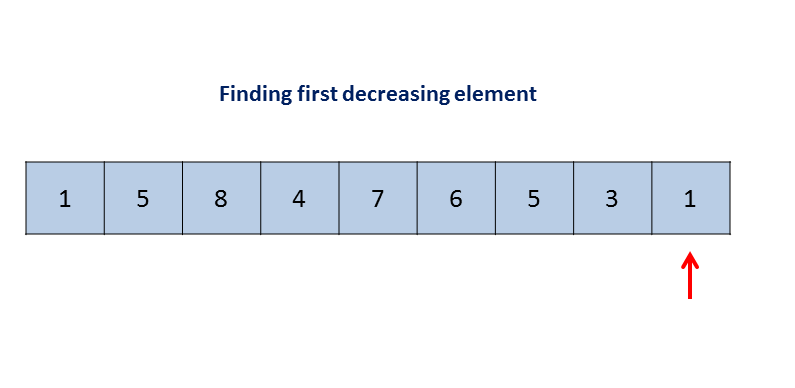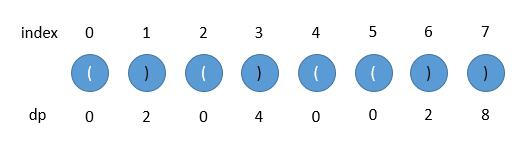# Next Permutation and Longest Valid Parentheses

Let’s continue…

Problem Statement:

Next Permutation

Implement next permutation, which rearranges numbers into the lexicographically next greater permutation of numbers.

If such arrangement is not possible, it must rearrange it as the lowest possible order (ie, sorted in ascending order).

The replacement must be in-place and use only constant extra memory.

Here are some examples. Inputs are in the left-hand column and its corresponding outputs are in the right-hand column.

`1,2,3``1,3,2`
`3,2,1``1,2,3`
`1,1,5``1,5,1`

Solution:

The description of this problem is a little bit tricky. I want to give more explanations. If you get the idea, you can skip this paragraph. In this problem, we will be given an integer array. All the elements of the array forms a number… for example, {1, 2, 6, 4, 5}, which means number 12645. Then we need to compute the next lager number which are formed by the permutation of the 5 numbers. To be more specific,  we need to find a specific permutation which is just greater than 12645 from all (5*4*3*2*1) permutations(in our case, it is 12654, no permutation between 12645~12654).

If we can not find a valid swap, we sort the array in ascending order.

Based on the interpretation, we have several tasks:

1. We need to swap elements from the array and make the newly formed number lager. Then we should swap larger element from the right of the array and the smaller element from the left. For example, {1,0,3} should be {1, 3, 0}. Only by that, we can make our number larger;
2. We only care about the next larger one,  so we should start from the right of the array, and check the first element we encounter which is smaller than the following element. When we find such an element i by searching from the end of the array to the i+1 element, and checking the smallest element among [i+1 : ] elements which is greater than i (it should exist one element which is greater than i, since i is the first element we encounter which is smaller than its right element i+1, at least we have i+1 element greater than i element).
3. When we find these two elements, we swap them. After the swap operation, the final number should be larger. However, this does not necessarily mean we find the next larger number. We still need to sort the [i+1 : ] elements in ascending order, so we can guarantee we get the target number.

Let’s look at a the progress in a dynamic image:Time Complexity: O(n) At most, we need to touch every element twice…

Space Complexity: O(1)

This file contains bidirectional Unicode text that may be interpreted or compiled differently than what appears below. To review, open the file in an editor that reveals hidden Unicode characters.

 class Solution { public: void nextPermutation(vector& nums) { int i = nums.size() – 2; while(i >= 0 && nums[i+1] <= nums[i]) i–; if(i>=0){ int j = nums.size() – 1; while(j >= i+1 && nums[j] <= nums[i]) j–; swap(nums[i],nums[j]); } reverse(nums.begin()+i+1, nums.end()); } };

Problem Statement:

Longest Valid Parentheses

Given a string containing just the characters `'('` and `')'`, find the length of the longest valid (well-formed) parentheses sub string.

Example 1:

```Input: "(()"
Output: 2
Explanation: The longest valid parentheses substring is `"()"````

Example 2:

`Input: "`)()())`" Output: 4 Explanation: The longest valid parentheses substring is `"()()"``

Solution One: Brute Force

A very straightforward solution. The general idea is that we check every sub string of the given string and find out whether they contain the valid parentheses, if they do contain the valid parentheses, we update the max length of the valid parentheses. If not, we move to the next sub string. I will not provide code here, it is pretty easy to write the code, however, the running time of the code is bad.

Time Complexity: O(n^3)

Space Complexity: O(n)

Solution Two: Optimized Brute Force

The Brute Force solution involves too much repetitive comparisons, we can get rid of them and improve the whole performance with some tricks. We also need to use two loops to iterate all possible sub strings, but this time, we apply another method to check whether the sub string is valid. We can use a counter and a stack to record how many ‘(‘ and ‘)’ we have already met when we iterate, if we meet ‘(‘, we increase the counter, and push this to the stack, when we encounter ‘)’, we decrease the counter and we pop the element from the stack. Whenever the counter reaches 0, we update the max length, and finally return that max length.

This optimized solution is much better compared to the original one.

Time Complexity: O(n^2)

Space Complexity: O(1)

This file contains bidirectional Unicode text that may be interpreted or compiled differently than what appears below. To review, open the file in an editor that reveals hidden Unicode characters.

 class Solution { public: int longestValidParentheses(string s) { int len_s = s.size(); int counter = 0, maxLen = 0; for(int i = 0; i < len_s; i++){ counter = 0; for(int j = i; j < len_s; j++){ if(s[j] == '('){ counter ++; } else{ counter –; } if(counter < 0) break; if(counter == 0){ maxLen = max(maxLen, j – i + 1); } } } return maxLen; } };

Solution Three: Dynamic Programming

When we want to apply DP approach, we should always consider the sub problem or sub questions.

The sub problem is: what is the length of the current longest (not total longest) valid parentheses when sub string ends at position i?

First thing we need to set the dp array all to 0. Since each dp[i] indicates the length of the current longest valid parentheses when sub string ends at position i, we need to consider several conditions:1)If the last element is ‘(‘, which means the sub string ends with left parenthesis, we remain the dp[i] = 0.  The reason we do this because the ‘(‘ is the starting position for all current sub string;

2) If the last element is ‘)’, we need to consider s[i-1]:

• If s[i-1] is ‘(‘, we can say we have to add dp[i-2] (the last length of the current longest valid parentheses) to 2 (current length is 2, one pair ‘()’), then we are done. I
• f the s[i – 1] is ‘)’, then we need to check the i – dp[i – 1] – 1, and see whether it is ‘(‘, if it is, we should add dp[i – 1], dp[i – dp[i- 1] – 2] and 2 together; if s[i – dp[i – 1] -1] is not ‘(‘, then we make dp[i] equal to 0.

It’s a little bit hard to describe, maybe I will update later with more pictures… Please look at the code below.

Time Complexity: O(n)

Space Complexity: O(n)

This file contains bidirectional Unicode text that may be interpreted or compiled differently than what appears below. To review, open the file in an editor that reveals hidden Unicode characters.

 class Solution { public: int longestValidParentheses(string s) { int len_s = s.size(); int dp[len_s+1]{0}; int maxLen = 0; for(int i = 1; i < len_s; i++){ if(s[i] == ')'){ if(s[i – 1] == '('){ dp[i] = (i-2>=0 ? dp[i – 2] : 0) + 2; } else{ if(i – dp[i-1] – 1 >= 0 && s[i – dp[i-1] – 1] == '(') dp[i] = dp[i-1] + 2 + (i-dp[i-1]-2 >=0 ? dp[i – dp[i-1] – 2] : 0); } } maxLen = max(maxLen, dp[i]); } return maxLen; } };

Solution Four : Stack

We can also use a stack to keep track of every step.

The general idea is:

we scan the whole string from left to right, whenever we encounter left parenthesis, we save the current position to our stack, then keep moving;

when we encounter a right parenthesis, we pop the top element of the stack, which means we have one right parenthesis paired with a left parenthesis, after that, we have two situations here:

1. If the stack is not empty, we deduct the current position with the current stack’s top value, then we get the current length of valid parentheses, we can update our maxLen variable;

2. If the stack is empty, which means we did not pair with any left parenthesis, we save current position to our stack.

Let’s run a demo:

• Initialize the stack, and we put -1 to the stack. Since the elements of the stack are all longest length of the valid parentheses, so they should all be positive. We can use -1 as the initialized value. We also need to maxLen to 0.• We encounter the left parenthesis first, we push the index to the stack.• Then we move forward, we can see that the next element is right parenthesis. We pop the top element. Since the stack is not empty, we need to calculate the current valid length, which is 1 – (-1) = 2. We update the maxLen variable with this value. We do not push this value to the stack, the stack should always store the index.• The next element is ‘)’ again, we need to pop the top element -1 out of the stack. We find that stack is empty now, which means this ‘)’ does not have any corresponding ‘(‘, we push the index of this element to the stack, which is 2.• Then we move forward, we meet left parenthesis again, we push the index 3, 4, 5 into the stack respectively.• The next is ‘)’ (index – 6), we need to pop the top element, and since stack is not empty, we need to calculate the current longest length (6 – 4 = 2) and update the maxLen.• The next element is ‘)’ again, we do the same thing, pop out 4, and calculate 7-3 = 4 and update the maxLen to 4.Let’s look at the code.

Time Complexity: O(n)

Space Complexity: O(n)

This file contains bidirectional Unicode text that may be interpreted or compiled differently than what appears below. To review, open the file in an editor that reveals hidden Unicode characters.

 class Solution { public: int longestValidParentheses(string s) { int len = s.length(); stack mySTK; mySTK.push(-1); int finalRes = 0; for(int i = 0; i < s.length(); i++){ int t = mySTK.top(); if(t != –1 && s[i] == ')' && s[t] == '('){ mySTK.pop(); finalRes = max(finalRes, i – mySTK.top()); } else{ mySTK.push(i); } } return finalRes; } };

Solution Five : Two pass scan

A very interesting solution. The general idea is we use two counters left and right to record the number of left parenthesis and right parenthesis respectively. When we iterate the string, when we encounter left parenthesis, we increase left, or we increase right. When right > left, we set both of them to 0, since we can not form any longer valid sub string any more, we have start counting again from the current position; when right == left, we update maxLen variable.

We have to iterate the string twice, one is from left to right and the other is right to left. The reason why we need to do this is to get rid of the special case. For example, “((())”, if we only iterate from left to right, we will never get valid answer.

Time Complexity: O(n)

Space Complexity: O(1)

This file contains bidirectional Unicode text that may be interpreted or compiled differently than what appears below. To review, open the file in an editor that reveals hidden Unicode characters.